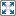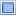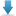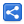# Python Variables

[Last Updated: Feb 1, 2022]

In Python we declare variables by assigning values:

`x = 1`

Python is not statically typed. It's dynamically typed.

We don't specify types when writing code or when declaring variables.

Python determines the types during runtime. It infers type by looking at the value.

In Python everything is object. Whenever we declare a variable, Python creates an object in the background.

## Declaring Variables

#### scripts/declaring-variables.py

```x = 1
print(x)
y = "test-string"
print(y)

```

#### Output

`1test-string`

## Implicit Type conversion

A variable which was previously assigned a value, can be assigned to another value of a different type. Python interpreter automatically converts variable's data type to another type if assigned a different type value:

#### scripts/implicit-type-conversion.py

```x = 1
print(x)
x = "test-string"
print(x)

```

#### Output

`1test-string`

## New memory allocation during implicit type change

When we declare variable, Python allocates a memory address and store the variable value in it.

When we change the variable value, Python allocates a new memory location.

The objects which are not referenced in the program are garbage collected (i.e. memory is deallocated by a background process).

In following example we are using Python's `id()` function which returns variable's memory address.

#### scripts/implicit-type-conversion-id.py

```x = 1
print(id(x))
x = "test-string"
print(id(x))

y = 1
print(id(y))
y = 2
print(id(y))
```

#### Output

`2791889174768279189424862427918891747682791889174800`

## Finding type of the variable

Python's `type()` function can be used to find the variable type:

#### scripts/finding-variables-type.py

```x = 1
print(type(x))
x = "test-string"
print(type(x))

```

#### Output

`<class 'int'><class 'str'>`

## Example Project

Dependencies and Technologies Used:

• Python 3.10.2
 Declaring Variables in PythonSelect AllDownload• python-variables
• scripts
• declaring-variables.py

 Share